# GMAT Math : Mixture Problems

## Example Questions

### Example Question #1 : Dsq: Understanding Mixture Problems

Two hydrochloric acid solutions, one of concentration 40% and one of concentration 25%, are mixed together to make a solution of 35% concentration.

1) 200 ml of 40% solution is used

2) 100 ml of 25% solution is used

Statement 2 ALONE is sufficient to answer the question, but Statement 1 ALONE is not sufficient.

BOTH statements TOGETHER are sufficient to answer the question, but NEITHER statement ALONE is sufficient to answer the question.

Statement 1 ALONE is sufficient to answer the question, but Statement 2 ALONE is not sufficient.

EITHER Statement 1 or Statement 2 ALONE is sufficient to answer the question.

BOTH statements TOGETHER are NOT sufficient to answer the question.

EITHER Statement 1 or Statement 2 ALONE is sufficient to answer the question.

Explanation:

Suppose we only know that 200 ml of the 40% solution is used. Then we can callthe amount of 25% solution used, and the total amount made is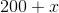. The solution equation becomes,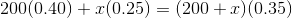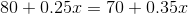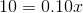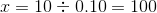So 100 ml of the 25% solution is used.

Similarly, if we only know that 100 ml of the 25% solution is used, then we can callthe amount of 40% solution used, and the total amount made is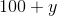. The solution equation becomes,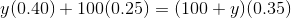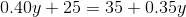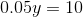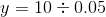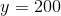So 200 ml of the 10% solution is used.

Either way, we get that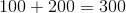and 300 ml of solution is created.

The answer is that either statement is sufficient to answer the question.

### Example Question #1 : Mixture Problems

A delivery truck is carrying two types of packages, green boxes and brown boxes. Green boxes weigh 10 lbs each, while brown boxes are 30 lbs each. At the weigh station, the driver notes that his load weighs exactly 400 lbs. How many green boxes does he have in his truck?

1. He is carrying exactly 20 boxes.

2. The driver never loads more brown boxes than green boxes.

Statement (1) ALONE is sufficient, but statement (2) alone is not sufficient to answer the question asked.

Statement (2) ALONE is sufficient, but statement (1) alone is not sufficient to answer the question asked.

BOTH statements (1) and (2) TOGETHER are sufficient to answer the question asked, but NEITHER statement ALONE is sufficient to answer the question asked.

Statements (1) and (2) TOGETHER are NOT sufficient to answer the question asked, and additional data specific to the problem are needed.

Statement (1) ALONE is sufficient, but statement (2) alone is not sufficient to answer the question asked.

Explanation:

Using statement 1, we can find that he has 10 green boxes and 10 brown. We solve mixture problems using 2 equations. If we letbe the number of green boxes, andbe the number of brown boxes. We can set the equations

1)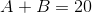and

2)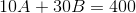Solving equation 1) for, we get.

Subsituting forinto equation 2) results in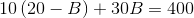Simplifying we get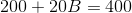or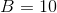Therefore, this answer would be 10 green boxes and 10 brown.

The second statement is irrelevant in finding our answer.

### Example Question #1 : Mixture Problems

Mark, the barista at Moose Jaw Coffee, has to mix together two kinds of coffee beans, Chocolate Explosion and Cherry Cherry Delight, to produce a blend that costs $12 per pound. How much of each coffee goes into the mixture? Statement 1: The Chocolate Explosion coffee costs$10 per pound.

Statement 2: The Cherry Cherry Delight coffee costs $16 per pound. Possible Answers: Statement 1 ALONE is sufficient to answer the question, but Statement 2 ALONE is NOT sufficient to answer the question. BOTH statements TOGETHER are insufficient to answer the question. Statement 2 ALONE is sufficient to answer the question, but Statement 1 ALONE is NOT sufficient to answer the question. EITHER statement ALONE is sufficient to answer the question. BOTH statements TOGETHER are sufficient to answer the question, but NEITHER statement ALONE is sufficient to answer the question. Correct answer: BOTH statements TOGETHER are insufficient to answer the question. Explanation: Assume both statements are true. Letbe the amount of Chocolate Explosion andbe the amount of Cherry Cherry Delight. Then the price of the Chocolate and Cherry coffees will be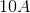dollars and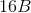dollars, respectively. The total price of the beans is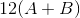, so we can set up the equation: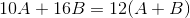However, there is no further information we can use to set up a second equation, so there is insufficient information to answer the question. ### Example Question #4 : Dsq: Understanding Mixture Problems Carl, the barista at Moose Jaw Coffee, has to mix together two kinds of coffee beans - Vanilla Heaven, which costs$10 a pound, and Mountain Goodness, which costs $15 a pound - to produce a blend that costs$12 a pound.

How many pounds of Vanilla Heaven coffee beans go into the mixture?

Statement 1: The mixture will include 30 pounds of Mountain Goodness beans.

Statement 2: The finished blend will weigh 75 pounds.

EITHER statement ALONE is sufficient to answer the question.

BOTH statements TOGETHER are insufficient to answer the question.

Statement 2 ALONE is sufficient to answer the question, but Statement 1 ALONE is NOT sufficient to answer the question.

BOTH statements TOGETHER are sufficient to answer the question, but NEITHER statement ALONE is sufficient to answer the question.

Statement 1 ALONE is sufficient to answer the question, but Statement 2 ALONE is NOT sufficient to answer the question.

EITHER statement ALONE is sufficient to answer the question.

Explanation:

Letbe the number of pounds of Vanilla Heaven coffee beans in the mixture.

If we assume Statement 1 alone, then the total price of the Vanilla Dream coffee beans is $10 per pound timespounds, or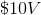. Similarly, the total price of the Mountain Goodness beans is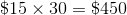, and the overall price of the beans in the mixture is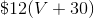. Add these together to get the equation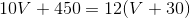. If we assume Statement 2 alone, the price of the Vanilla Dream beans is again. However, the price of the Mountain Goodness beans is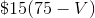and the overall price of the beans is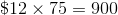, so we can set up this equation: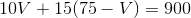From either equation we can solve forand get the answer to the question. ### Example Question #5 : Dsq: Understanding Mixture Problems A chemist dilutesliters of aacid solution with water to obtainliters of aacid solution. How many liters of water must be added to the original acid solution? (1)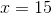liters (2)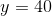liters Possible Answers: Both statements TOGETHER are sufficient, but NEITHER statement ALONE is sufficient Both statements TOGETHER are not sufficient. Statement (2) ALONE is sufficient, but Statement (1) ALONE is not sufficient Statement (1) ALONE is sufficient, but Statement (2) ALONE is not sufficient Each statement ALONE is sufficient. Correct answer: Each statement ALONE is sufficient. Explanation: Using Statement (1), we can set up the following equation: The amount of acid in the original solution (40% multiplied by 15 liters) should equal the amount of acid in the final diluted solution (remember there is no acid in water!!!)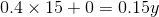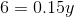Therefore knowing the value of x, we are able to calculate y. We can now find the amount of water used to dilute the solution by subtracting x from y.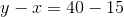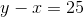Using Statement (2), we set up the following equation: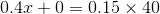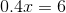We can also calculate the amount of water used since we can calculate x from knowing y.Each Statement ALONE is sufficient to answer the question. ### Example Question #2191 : Gmat Quantitative Reasoning How much should a grocer sell a mixture of almonds and walnuts for? (1) A pound of almonds is twice as expensive as a pound of walnuts. (2) The mixture contains 0.7 pounds of walnuts and 0.3 pounds of almonds. Possible Answers: Each Statement ALONE is sufficient. Both statements TOGETHER are sufficient, but NEITHER statement ALONE is sufficient. Statement (1) ALONE is sufficient, but Statement (2) ALONE is not sufficient. Statements (1) and (2) TOGETHER are not sufficient. Statement (2) ALONE is sufficient, but Statement (1) ALONE is not sufficient. Correct answer: Statements (1) and (2) TOGETHER are not sufficient. Explanation: (1) A pound of almonds is twice as expensive as a pound of walnuts. Let x be the price per pound of walnuts and y the price per pound of almonds.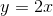We don't know the respective amounts of almonds and walnuts and the prices of any of the nuts aren't clearly stated. Statement(1) Alone is not sufficient. (2) The mixture contains 0.7 pounds of walnuts and 0.3 pounds of almonds. Statement (2) alone is not sufficient, it indicates the quantities of almonds and walnuts in a pound of mixture. However the price per pound of each type of nuts is unknown. Combining both statements, we can write the price of one pound of the mixture as: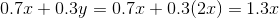We do not know x, the price per pound of walnuts, therefore both statements together are not sufficient. ### Example Question #1 : Mixture Problems What is the price of a pound of almonds? (1) A mixture of half almonds and half walnuts sells for$14 per pound.

(2) A pound of almonds is twice as expensive as the pound of walnuts.

Both statements TOGETHER are sufficient, but NEITHER statement ALONE is sufficient.

Statement (2) ALONE is sufficient, but Statement (1) ALONE is not sufficient.

Statement (1) ALONE is sufficient, but Statement (2) ALONE is not sufficient.

Each Statement ALONE is sufficient.

Statements (1) and (2) TOGETHER are not sufficient.

Both statements TOGETHER are sufficient, but NEITHER statement ALONE is sufficient.

Explanation:

(1) A mixture of half almonds and half walnuts sells for \$14 per pound.

Let x be the price per pound of walnuts and y the price per pound of almonds.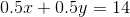Since we do not know the price per pound of walnuts or the price per pound of almonds, statement(1) is not sufficient.

(2) A pound of almonds is twice as expensive as the pound of walnuts.

Let x be the price per pound of walnuts and y the price per pound of almonds.Statement (2) alone is not sufficient.

Combining both statements: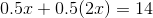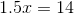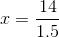The price per pound of almonds is: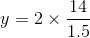### Example Question #8 : Dsq: Understanding Mixture Problems

How much water does a chemist need to dilute a pure solution of chlorine in order to obtain 150 ml of solution?

(1) The chemist would like the final solution to be 15% chlorine.

(2) There is no chlorine in water.

Each Statement ALONE is sufficient.

Statement (2) ALONE is sufficient, but Statement (1) ALONE is not sufficient.

Statements (1) and (2) TOGETHER are not sufficient.

Both statements TOGETHER are sufficient, but NEITHER statement ALONE is sufficient.

Statement (1) ALONE is sufficient, but Statement (2) ALONE is not sufficient.

Statement (1) ALONE is sufficient, but Statement (2) ALONE is not sufficient.

Explanation:

(1) The chemist would like the final solution to be 15% chlorine.

The final solution is 150 ml and contains 15% of chlorine. Let x be the amount of water, then 150-x is the amount of the original chlorine solution. The amount of chlorine in the original solution is the same in the final solution, only the concentration changes.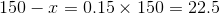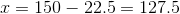(2) There is no chlorine in water.

Statement (2) is not helpful as it does not help in finding the amount of chlorine in the final solution.

### Example Question #2191 : Gmat Quantitative Reasoning

What quantity of solution is obtained by dilutingliters of pure acid with water?

(1) The final solution contains 20% of acid.

(2)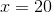ml.

Both statements TOGETHER are sufficient, but NEITHER statement ALONE is sufficient.

Statements (1) and (2) TOGETHER are not sufficient.

Statement (2) ALONE is sufficient, but Statement (1) ALONE is not sufficient.

Statement (1) ALONE is sufficient, but Statement (2) ALONE is not sufficient.

Each Statement ALONE is sufficient.

Both statements TOGETHER are sufficient, but NEITHER statement ALONE is sufficient.

Explanation:

(1) The final solution contains 20% of acid.

Using Statement (1), we know the concentration of acid in the final solution. However, we cannot find the quantity of the final solution as we do not know what quantity of acid was diluted.

So Statement (1) Alone is not sufficient.

(2) x=20 ml

Using statement (2) we know the quantity of the original acid solution, but we do not know what quantity of water was added or the concentration of the final solution.

So Statement (2) Alone is not sufficient.

Combining both Statements,

We have 20 ml of a 100% acid solution. Note that there is 0% acid in water. After diluting the solution, we obtain y ml of a solution containing 20% of acid. The amount (in ml) of acid in the final solution equals the amount of acid of the initial solution: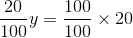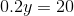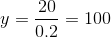Therefore 100 ml of solution is obtained by diluting the original acid solution with water.

Both Statements Together are sufficient.

### All GMAT Math Resources